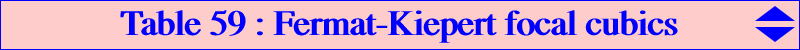Consider the two following decomposed cubics : • (K1) is the union of the line at infinity and the Kiepert hyperbola. • (K2) is the union of the Fermat axis and the orthocentroidal circle. Their common points are X(2), X(4), X(13), X(14), X(542), the circular points at infinity denoted J1 and J2, the common points S1 and S2 of the Kiepert hyperbola, the orthocentroidal circle, the perpendicular bisector of the Fermat points. The four latter points are always imaginary. (K1) and (K2) generate a pencil of cubics passing through these nine points and all are focal cubics with singular focus F on the Euler line. If (KF) denotes the cubic of the pencil with focus F (hence passing through F) then (KF) can be seen as the locus of contacts of tangents drawn through F to the circles passing through the Fermat points. Obviously, the circle that passes through F is the polar conic (C) of F. Note that, if F ' is the second point of this latter circle with the Euler line then the cubics (KF) and (KF ') share the same circular polar conic. See the pair of Fermat-Lester cubics K871, K872 for example. F and F ' are inverses in the orthocentroidal circle hence they coincide when F = X(2) or F = X(4) giving the cubics K874, K875 which are the only cubics not in pairs. It follows that the orthic line (sometimes called axis) of the focal is the Fermat axis and (KF) is therefore unipartite. The real asymptote is the homothetic of the Fermat axis under h(F, 2) and this asymptote meets the focal again at X which lies on the hyperbola passing through X(2), X(4), X(6), X(523), X(542). The parallel at F to the Fermat axis meets the focal again at Y, also on the perpendicular bisector of the Fermat points namely the line X(115)X(125). All this results from well known properties of focal cubics. *** Third points and consequences Let us denote by • Q1, Q2 the third points of (KF) on the lines passing through X(2) and X(13), X(14) resp. • Q3, Q4 the third points of (KF) on the lines passing through X(4) and X(13), X(14) resp. • Q5, Q6 the third points of (KF) on the lines passing through X(542) and X(2), X(4) resp. Recall that X(13), X(14), X(542) are collinear points and a lot of other collinearities are easily established : • X(13), Q2, Q6 and X(13), Q4, Q5 • X(14), Q1, Q6 and X(13), Q3, Q5 • F, Q5, Q6 • X, Q1, Q3 and X, Q2, Q4 • Y, Q1, Q4 and Y, Q2, Q4. This is related to the usual conjugation on a focal cubic. For example, {X(2), Q6}, {X(4), Q5}, {Q1, Q2}, {Q3, Q4} are pairs of conjugated points and two points of a pair share the same tangential. See figure 3. *** Related loci Recall that F lies on the Euler line and Y lies on the perpendicular bisector of the Fermat points. When F traverses the Euler line, • the locus of X is the hyperbola (H) passing through X(2), X(4), X(6), X(523), X(542). See figure 1. • the envelope of the line passing through Q1, Q2 is the parabola (P1) with focus X(6792), directrix the line X(4)X(2793), passing through X(115), X(543), X(7737). • the envelope of the line passing through Q3, Q4 is the parabola (P2) with focus X(6794), directrix the line X(2)X(2799), passing through X(115), X(2549), X(2794). Note that (P1) and (P2) are both tangent at X(115) to the Fermat axis and also both tangent to the line X(6)X(60) at X(7737), X(2579) respectively. (P1) is also tangent to the lines X(2)X(13), X(2)X(14), X(381)X(542). The foci X(6792), X(6794) of (P1), (P2) are two points on the orthocentroidal circle. See figure 2. • the envelope of the line passing through Q5, Q6 is the ellipse (E) with foci X(13) and X(14), with center X(115), passing through X(1316) with tangent the Euler line and through X(125) with tangent passing through X(2). The Fermat axis and the perpendicular bisector of the Fermat points are the axes of (E). See figure 3. • the locus of the centers of anallagmaty (two are real, two are imaginary) is a cubic (K) passing through X(2), X(4), X(542), X(868), the infinite points of the rectangular circum-hyperbola passing through X(99). Hence, (K) has three real asymptotes and two of them pass through X(868). See figure 4.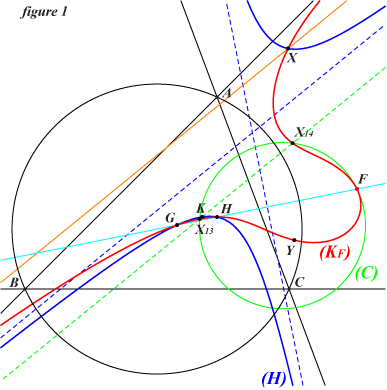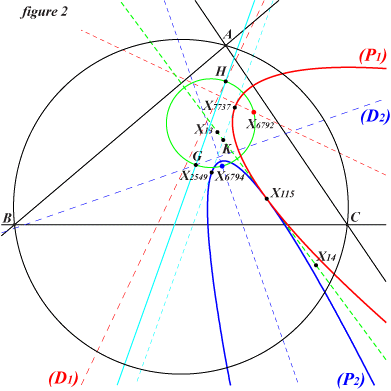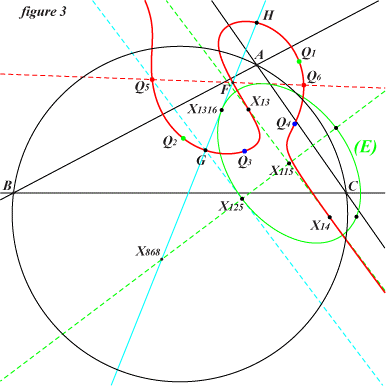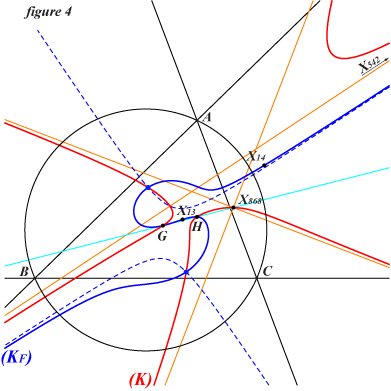The following table gives a selection of Fermat-Kiepert focal cubics according to the singular focus F on the Euler line. Each cubic passes through the nine fixed points X(2), X(4), X(13), X(14), X(542), J1, J2, S1, S2 mentioned at the top of this page and not repeated in the table. The points X, Y are given when possible. The polar conic (C) of F is a circle passing through X(13), X(14), F not repeated. Two cubics highlighted with the same color share the same polar conic (C).cubic F X Y other centers on the cubic centers on (C) remark K870 X(14694) X(14832) X(1648) X(111), X(14694), X(14833), X(14834) K871 X(3) X(15356) X(15357) X(5), X(1117) (C) = Lester circle K872 X(5) X(15358) X(15359) X(15342) X(3), X(1117) (C) = Lester circle K873 X(868) X(542) X(868) X(9140) X(1316), X(1640) axial cubic K874 X(2) X(4) X(125) X(14847) X(111), X(476) (C) = Hutson-Parry circle K875 X(4) X(2) X(16278) X(2132), X(2394) K876 X(1316) X(16279) X(16280) X(10653), X(10654) X(1640) K966 X(16281) 0.925436658135907 X(16282) X(69), X(148), X(5108)